Power Torque continues to be a leading integrator in the motion control and electrical / mechanical power transmission fields. Let our combined experience of over 400 years design a solution for you.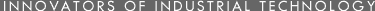• •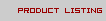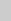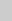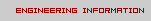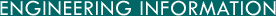A B C D E F G

A

• Acre (US)
= 0.0015625 square miles, 10 square chains (Gunter's), 160 square rods (square perches), 4840 square yards, 43,560 square feet, 100,000 square links (Gunter's), 0.4046873 hectares (square hectometers), 40.46873 ares (square dekameters), 4046.873 square meters.

• Acre (Br)
= 4 Br roods, 4840 square Br yards, 0.4046849 hectares (square hectometers), 4046.849 square meters.

• Acre Foot (US)
= 43,560 cubic feet, 1,613.33 cubic yards, 325,872 gallons, 1233.5 cubic meters, 1,233,489 liters.

Acres inches = 27,154 gallons.

Acres inch per hour = 452 GPM.

Angstrom Unit = 3.937x10^-9 inch, 0.003937 millionths of an inch, 1x10^-10 meters, 1x10^-8 centimeters, 1x10^-4 microns, 0.1 milli-micron (micro-millimeter), 100 millionth microns (micro-microns).

Are = 3.8610x10^-5 square mile, 0.02471044 acre, 119.60 square yards, 1076.4 square feet, 0.01 hectare, 1 square dekameter, 100 square meters.

Astronomical Unit = 1.495x10^8 kilometers, 0.48481x10^-5 parsecs, 1.5812x10^-5 light years.

Atmosphere (pressure) = Pressure exerted by 76 cm of Hg at density 13.5951 grams per cubic centimeter at G=980.665 centimeters per square second, 0.0073480 ton (2000 pound) per square inch, 1.0133 bars, 1.0581 tons (2000 pound) per square foot, 14.696 pounds per square inch, 29.921 inches of Hg at 32 degrees F, 33.899 feet of water at 39.1 degrees F, 760 millimeters of Hg at 0 degrees C, 1033.2 grams per square centimeter, 2116.2 pounds per square foot, 1.0332x10^4 kilograms per square meter, 1.01325x10^6 dynes per square centimeter, 101.325 kPascal.

B

• Bag (Br) = 3 dry bushels, 0.109107 cubic meter.

• Bar (pressure)
= 0.98692 atmosphere, 14.504 pounds per square inch, 1.01971x10^4 kilograms per square meter, 1.000x10^6 dynes per square centimeter.

• Barye (pressure)
= 1 dyne per square centimeter.

• Barleycorn (Br)
= 1/3 inch, 0.84667 centimeters.

• Barn
= 1x10-3 radians, 1x10^-24 square centimeters.

• Barrel (US dry)
= 3.281 bushels, 105.0 dry quarts, 7056 cubic inches except cranberry barrel which equals 5826 cubic inches, 0.11562 cubic meter.

Barrel (US liquid) = 42 gallons (oil), 31.5 gallons (beer), 30.0 gallons (wine), 0.11924 cubic meter.

Barrel (Br dry) = 36 Br gallons, 0.16366 cubic meter.
Board Foot = 1/12 cubic foot, 144 cubic inches (1 ft x 1 ft x 1 in), 2359.8 cubic centimeters.

Bolt (US Cloth) = 120 linear feet, 36.576 meters.
British Thermal Unit (BTU, mean) = 0.25198 kilogram calorie (large mean calorie), 777.97 foot pounds, 3.9292x10^-4 horsepower hour, 2.930x10^-4 Kilowatt hour, 0.2930 watt hour, 10.409 liter atmospheres, 107.56 kilogram meters, 251.98 mean gram calories, 1054.8 absolute joules, 0.3676 cubic foot atmospheres, 2.5030x10^4 foot poundals, 1.0548x10^10 ergs.

BTU (39 degrees F) = 1060.4 absolute joules.

BTU (60 degrees F) = 1054.6 absolute joules.

BTU per Minute = 12.96 foot pounds per second, 0.02356 horsepower, 0.01757 kilowatts, 17.57 watts.

Bucket (Br dry) = 4 Br gallons, 1.8184x10^4 cubic centimeters.

Bushel (US dry) = 0.304785 barrel, 0.96895 Br bushel, 1.2444 cubic feet, 4 pecks, 32 dry quarts, 64 dry pints, 2150.42 cubic inches, 0.035239 cubic meter, 0.35238 hectoliter, 3.5238 dekaliters, 35.238 liters, 3.5239x10^4 cubic centimeters.

Bushel (Br dry) = 1/8 Br quarter, 1/3 Br bag, 1.03205 bushels, 1.2843 cubic feet, 8 Br gallons, 2219.3 cubic inches, 0.363677 hectoliter, 3.63677 dekaliters, 36.3677 liters, 3.6369x10^4 cubic centimeters.

Butt (Br dry) = 126 gallons, 0.57281 cubic meter.

C

• Cable Length = 720 feet, 219.46 meters.
Calorie - see gram calorie or kilogram calorie.

• Carat
= 3.08647 grains, 205.6 milligrams.

• Cental
= 100 pounds, 45.359 kilograms.

• Centare
= 1.196 square yards, 10.764 square feet, 1550 square inches, 0.01 are, 1 square meter.

• Centigram
= 0.1543236 grain, 0.01 gram.

• Centiliter
= 0.33815 fluid ounce, 0.61025 cubic inch, 2.705179 fluid drams, 0.01 liter, 10.000027 cubic centimeters.

• Centimeter
= 0.01093611 yard, 0.01093614 Br yard, 0.032808 foot, 0.39370 inch, 4.4330 linges (Paris lines), 393.70 mils, 0.01 meter, 10 millimeters, 1x10^4 microns, 1x10^7 milli-microns (micro-millimeters)1x10^8 Angstroms.

• Centimeter-dyne
= See erg.

• Centipoise (viscosity)
= 0.01 poise, centistokes x density at a given temperature.

• Centistoke (viscosity)
= 0.01 stoke.

• Chain (Engineer's or Ramden's)
= 100 feet, 100 links of 1 foot, 30.4801 meters.

• Chain (Surveyor's or Gunter's)
= 0.1 furlong, 0.0125 mile, 4 rods, 22 yards, 66 feet, 100 links, 792 inches, 20.117 meters, 2011.7 centimeters.

• Chaldron (US dry)
= 36 bushels (variable), 1.2686 cubic meters.

• Chaldron (Br dry)
= 32 Br bushels (variable), 1.1638 cubic meters.

• Cheval-vapeur
= for electrical purposes, usually 736 watts.

• Cheval-vapeur heure
= 2.6478x10^6 joules (absolute).

• Circle
= 2 Pi, 6.28319 radians, 12 signs, 360 degrees.

• Circular inch
= area of a circle the diameter of which is one inch, 0.78540 square inch, 5.0671 square centimeters.

• Circular mil
= area of a circle the diameter of which is one mil or 1/1000 inch, 7.854x10^-7 square inch, 0.78540 square mil, 5.0671 square centimeter, 5.0671x10^-4 square millimeter.

• Circular millimeter
= area of a circle the diameter of which is one millimeter, 0.0078450 square centimeter, 0.78540 square millimeter.

• Circumference
= 2 Pi, 6.28319 radians, 360 degrees, 400 grades, 2.1600x10^4 minutes, 1.296000x10^6 seconds.

• Clove or customary stone
(Br) = 8 pounds, 3.6287 kilograms.

• Coomb
(Br dry) = 4 bushels, 0.14548 cubic meter.

• Cord
= 8 cord feet, 128 cubic feet (8 x 4 x 4), 3.625 cubic meters.

• Cord foot
= 1/8 cord, 16 cubic feet (4 x 4 x 1).

• Cubic centimeter
= 1.3079x10^-6 cubic yard, 2.83776x10^-5 bushel, 2.7496x10^-5 Br bushel, 3.531445x10^-5 cubic foot, 3.531477x10^-5 Br cubic foot, 2.6417x10^-4 gallon, 2.1997x10^-4 Br gallon, 4.2376x10^-4 board foot, 0.0010567 liquid quart, 8.7988x10^-4 liquid Br quart, 9.0808x10^-4 dry quart, 0.0021134 liquid pint, 0.0018162 dry pint, 0.033814 fluid ounce, 0.035195 fluid Br ounce, 0.061023 cubic inch, 0.27051 fluid dram, 0.38257 fluid Br drachm, 16.231 minims, 16.894 Br minims, 1x10^-6 cubic meter, 9.9997x10^-4 liter, 0.001 cubic decimeter, 0.99997 milliliter, 1000 cubic millimeters.

• Cubic centimeter-atmosphere (normal)
= 0.101325 joule (absolute).

• Cubic Decimeter
= 0.0013079 cubic yard, 0.035314 cubic foot, 61.023 cubic inches, 0.001 cubic meter, 0.99997 liter, 1000 cubic centimeters.

• Cubic Dekameter
= 1000 cubic meters.

• Cubic Foot (US)
= 1/128 cord, 0.01 Br register ton, 1/27 cubic yard, 1/16 cord-foot, 0.80357 bushel, 0.77861 Br bushel, 7.481 gallons, 6.229 Br gallons, 12 board feet, 25.714 dry quarts, 29.922 liquid quarts, 59.844 liquid pints, 1728 cubic inches, 0.02831701 cubic meter, 28.316 liters, 2.8317x10^4 cubic centimeters.

• Cubic foot (BR)
= 0.02831677 cubic meter, 2.831677x10^4 cubic centimeters.

• Cubic foot (of water)
= 62.4 pounds, 999.8 ounces, 28.315 kilograms.

• Cubic foot-atmosphere
= 2.7203 BTU (mean), 28.313 liter-atmosphere, 292.59 kilogram-meters, 680.74 gram-calories (mean), 2116.3 foot pounds, 2869.4 joules (absolute).

• Cubic inch (US)
= 2.143347x10^-5 cubic yard, 4.65025x10^-4 bushel, 5.78704x10^-4 cubic foot, 0.00186010 peck, 0.0043290 gallon, 1/144 board foot, 0.014881 dry quart, 0.017316 liquid quart, 0.0297616 dry pint, 0.5541 fluid ounce, 4.4329 fluid drams, 1.6387162x10^-5 cubic meter, 0.0163868 liter, 1.63868 centiliters, 16.3868 milliliters, 16.387162 cubic centimeters, 1.6387162x10^4 cubic millimeters.

• Cubic inch (Br)
= 4.5081x10^-4 Br bushel, 5.7870x10^-4 Br cubic foot, 0.0018031 Br peck, 0.003606 Br gallon, 16.3870253 cubic centimeters.

• Cubic Hectometer
= 1x10^6 cubic meters.

• Cubic Kilometer
= 1x10^9 cubic meters.

• Cubic Meter
= 0.2759 cord, 1.3079428 cubic yards, 1.307954 cubic Br yards, 28.3776 bushels, 35.314445 cubic feet, 35.31477 Br cubic feet, 264.173 gallons, 1056.7 liquid quarts, 2113.4 liquid pints, 6.1023x10^4 cubic inches, 1 stere, 999.973 liters, 1000 cubic decimeters, 1x10^6 cubic centimeters, 1x10^9 cubic millimeters.

• Cubic Millimeter
= 6.1023x10^-5 cubic inch, 0.01623 minim, 0.01689 BR minim, 1x10^-9 cubic meter, 0.001 cubic centimeter.

• Cubic Yard
(US) = 27 cubic feet, 202.0 gallons, 168.17 Br gallons, 807.9 liquid quarts, 1616 liquid pints, 4.6656x10^4 cubic inches, 0.76455285 cubic meter, 764.54 liters, 7.6455945x10^5 cubic centimeters.

• Cubic Yard
(Br) = 27 cubic feet, 0.76455945 cubic meter.

• Cubit
= 18 inches, 45.72 centimeters.

• Cubit
(Royal) = 25 inches, 63.50 centimeters

D

• Dalton = 1/16 the mass of an atom of oxygen, 1.650x10^-24 gram.

• Day (tropical mean solar)
= 24 mean solar hours, 1440 mean solar minutes, 8.6400X10^4 mean solar seconds.

• Day (sidereal)
= 8.6164x10^4 mean solar seconds.

• Decigram
= 1.543236 grains, 0.1 gram.

• Deciliter
= 0.176 BR pint, 3.38147 fluid ounces, 0.1 liter, 100.0027 cubic centimeter.

• Decimeter
= 0.3280833 foot, 0.3280843 BR foot, 3.93700 inches, 3.937011 BR inches, 0.1 meter.

• Decistere
= 0.1 stere (cubic meter).

• Degree (angle)
= 1/360 circumference (revolution), 1/90 quadrant, 0.017453 radian, 60 minutes, 3600 seconds.

• Degree (F)
= 9C/5+32

• Degree (C)
= 5F/9-17.7778

• Degree (absolute, K)
= C+273.15

• Dekagram
= 0.35273957 avoirdupois ounce, 5.64383 avoirdupois drams, 0.01 kilogram, 10 grams.

• Dekaliter
= 0.28378 bushel, 0.27497 Br bushel, 1.13513 pecks, 9.08102 dry quarts, 18.162 dry pints, 10 liters, 10.00027 cubic decimeters.

• Dekameter
= 1.98838 rods, 10.93611 yards, 10.93614 Br yards, 393.70 inches, 10 meters.

• Dekastere
= 10 steres (cubic meters).

• Drachm (fluid Br)
= 1/8 fluid Br ounce, 60 minims, 3.5515 cubic centimeters.

• Dram (apothecaries or troy)
= 1 Br drachm, 0.008571429 avoirdupois pound, 1/96 apothecary or troy pound, 0.1371429 avoirdupois ounce, 1/8 apothecary or troy ounce, 2.194286 avoirdupois drams, 2.5 pennyweights, 3 scruples, 60 grains, 3.8879351 grams.

• Dram (avoirdupois)
= 0.0047471788 apothecary or troy pound, 1/256 avoirdupois pound, 0.056966146 apothecary or troy ounce, 0.0625 avoirdupois ounce, 0.4557292 apothecary or troy dram, 1.139323 pennyweights, 1.3671875 scruples, 27.34375 grains, 1.771845 grams.

• Dram (fluid)
= 0.00390625 liquid quart, 0.0078125 liquid pint, 0.03125 gill, 1/8 fluid ounce, 0.225586 cubic inch, 60 minims, 3.6966 milliliters, 3.6967 cubic centimeters.

• Dyne (force)
= 2.2481x10^-6 pound weight, 7.2330x10^-5 poundal, 0.0010197 gram weight, 0.015737 grain weight. (Assumes G=980.665 cm / second squared).

E

• Ell = 45 inches, 114.30 centimeters.

• Em, Pica
= 1/6 inch, 0.42333 centimeter.

• Erg
= 2.3889x10^-11 kilogram calorie (mean), 9.4805x10^-11 BTU (mean), 1.0197x10^-8 kilogram-meter, 2.3889x10^-8 gram calorie (mean), 7.3756x10^-8 foot pound, 1x10^-7 joule, 2.3730x10^6 foot poundal, 0.0010197 gram centimeter, 1 dyne-centimeter.

F

• Fathom = 6 feet, 1.828804 meters.

• Fermi
= 1x10^-15 meter.

• Firkin
= 9 gallons, 34.068 liters.

• Foot (US)
= 1.645788x10^-4 nautical mile, 1.893939x10^-4 statute mile, 0.00151515 furlong, 0.0151515 Gunter's chain, 0.0606061 rod, 1/6 fathom, 1/3 yard, 12 inches, 0.3048006 meter, 30.48006 centimeters, 473404 wavelengths of red line of cadmium.

• Foot (Br)
= 0.4 Br pace, 30.47997 centimeters.

• Foot (Paris)
= 1 Pied.

• Foot of water (pressure) at 4 degrees C
= 0.029499 atmosphere, 0.43352 pound per square inch, 0.88265 inch of Hg at 32 degrees F, 62.426 pounds per square foot, 304.79 kilograms per square meter.

• Foot Pound
= 3.7662x10^-7 kilowatt-hour, 5.0505x10^-7 horsepower-hour, 3.2389x10^-4 kilogram-calorie (mean), 3.7662x10^-4 watt-hour, 4.7253x10^-4 cubic foot-atmosphere, 0.0012854 BTU (mean), 0.013381 liter-atmosphere, 0.138255 kilogram-meter, 0.32389 gram-calorie (mean), 1.35582 joule (absolute), 32.174 foot poundals, 1.3825x10^4 gram centimeters, 1.35582x10^7 ergs.

• Foot Pondal
= 3.9952x10^-5 BTU (mean), 4.1589x10^-4 liter-atmosphere (normal), 0.0042972 kilogram-meter, 0.010067 gram-calorie, 0.031081 foot-pound, 0.042140 joule, 4.21402x10^5 ergs.

• Furlong (US or Br)
= 1/8 mile, 10 Gunter's chains, 40 rods, 220 yards, 660 feet, 201.168 meters.

G

• Gallon (US) = at 62 degrees F weighs 3.7820 kilogram or 8.337 avoirdupois lbs, 0.004951 cubic yard, 0.031746 liquid barrel, 0.13368 cubic foot, 0.83268 Br gallon, 4 quarts, 8 pints, 32 gills, 128 ounces, 231.00 cubic inches, 6.144x10^4 minims, 0.0037854 cubic meter, 3.7853 liters, 3785.4 cubic centimeters.

• Gallon (Br)
= at 62 degrees F weighs 10 avoirdupois pounds, 0.02778 dry Br barrel, 1/8 dry Br bushel, 0.16054 cubic foot, 0.5 Br peck, 1.20094 gallons, 4 Br quarts, 8 Br pints, 32 Br gills, 160 Br ounces, 277.3 cubic inches, 4.54596 liters, 4545.96 cubic centimeters.

• Geepound
= See slug.

• Gill (US)
= 1/32 gallon, 1/8 quart, ¬ pint, 4 fluid ounces, 7.21875 cubic inches, 32 drams, 1920 minims, 0.118292 liters, 118.295 cubic centimeters.

• Gill (Br)
= 1/32 Br gallon, ¬ Br pint, 5 fluid Br ounces, 0.14206 liter, 142.07 cubic centimeters.

= 1/400 circumference, 0.0157079 radian, 0.9 degree, 100 centesimal minutes.

• Grain
= 1/7000 avoirdupois pound, 1/5760 apothecary or troy pound, 0.0022857 avoirdupois ounce, 0.0020833 apothecary or troy ounce, 0.03657143 avoirdupois dram, 0.016667 apothecary or troy dram, 0.0416667 troy pennyweight, 0.05000 apothecary scruple, 0.064798918 gram, 0.3240 metric carat, 64.798918 milligram.

• Gram
= 0.00220462 avoirdupois pound, 0.00267923 apothecary or troy pound, 0.0352739 avoirdupois ounce, 0.0321507 apothecary or troy ounce, 0.564383 avoirdupois dram, 0.257206 apothecary or troy dram, 0.6430149 pennyweight, 0.771618 scruple, 15.4324 grains, 1x10^-6 metric ton, 1x10^-4 myriagram, 0.001 kilogram, 5 metric carats, 1000 milligrams, 1x10^6 microgram.

• Gram-Calorie (mean)
= 1.5593x10^-6 horse power hours, 0.001 kilogram calorie, 0.0011628 watt-hour, 0.001459 cubic foot atmosphere, 0.0039685 BTU (mean), 0.041311 liter-atmosphere, 0.42685 kilogram-meter, 3.0874 foot-pounds, 4.186 joules (absolute), 99.334 foot-poundals.

• Gram-calorie (15°C)
= 4.185 joules (absolute).

• Gram-calorie (20°C)
= 4.181 joules (absolute).

• Gram-centimeter
= 2.3427x10^-8 kilogram-calorie (mean), 9.2972x10^-8 BTU (mean), 1x10^-5 kilogram-meter, 2.3427x10^-5 gram-calorie (mean), 7.233x10^-5 foot-pound, 9.80665x10^-5 joule (absolute), 980.7 ergs.